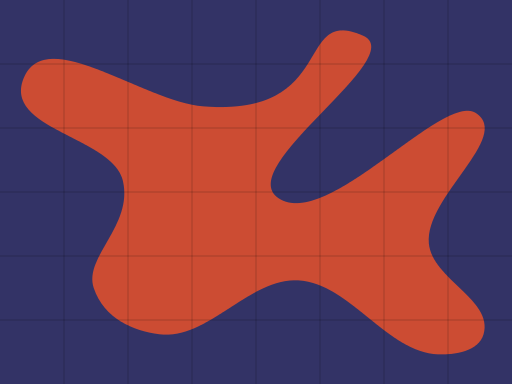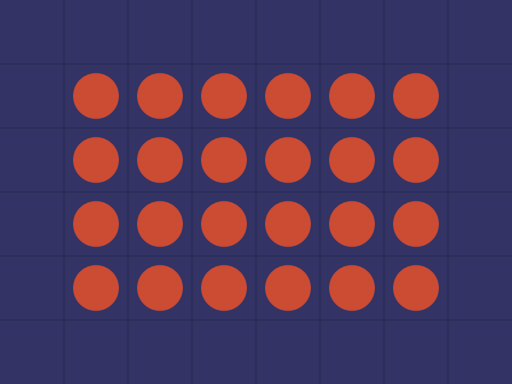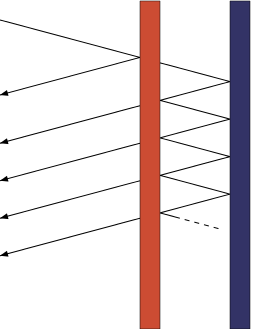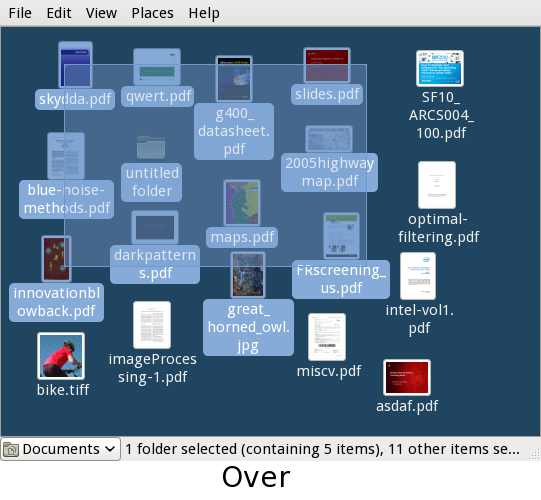# Over is not Translucency

The Porter/Duff Over operator, also known as the “Normal” blend mode in Photoshop, computes the amount of light that is reflected when a pixel partially covers another:The fraction of bg that is covered is denoted alpha. This operator is the correct one to use when the foreground image is an opaque mask that partially covers the background:A photon that hits this image will be reflected back to your eyes by either the foreground or the background, but not both. For each foreground pixel, the alpha value tells us the probability of each:

$a \cdot \text{fg} + (1 - a) \cdot \text{bg}$

This is the definition of the Porter/Duff Over operator for non-premultiplied pixels.

But if alpha is interpreted as translucency, then the Over operator is not the correct one to use. The Over operator will act as if each pixel is partially covering the background:Which is not how translucency works. A translucent material reflects some light and lets other light through. The light that is let through is reflected by the background and interacts with the foreground again.Let’s look at this in more detail. Please follow along in the diagram to the right. First with probability $a$, the photon is reflected back towards the viewer:

\displaystyle \begin{align*} &a \cdot \text{fg} \end{align*}

With probability $(1 - a)$, it passes through the foreground, hits the background, and is reflected back out. The photon now hits the backside of the foreground pixel. With probability $(1 - a)$, the foreground pixel lets the photon back out to the viewer. The result so far:

\displaystyle \begin{align*} a\cdot \text{fg} &+(1 - a) \cdot \text{bg} \cdot (1 - a) \end{align*}

But we are not done yet, because with probability $a$ the foreground pixel reflects the photon once again back towards the background pixel. There it will be reflected, hit the backside of the foreground pixel again, which lets it through to our eyes with probability $(1 - a)$. We get another term where the final $(1 - a)$ is replaced with $a \cdot \text{fg} \cdot \text {bg} \cdot (1 - a)$:

\displaystyle \begin{align*} a\cdot \text{fg} &+(1 - a) \cdot \text{bg} \cdot (1 - a)\\ &+(1 - a) \cdot \text{bg} \cdot a \cdot \text{fg} \cdot \text{bg} \cdot (1 - a) \end{align*}

And so on. In each round, we gain another term which is identical to the previous one, except that it has an additional $a \cdot \text{fg} \cdot \text{bg}$ factor:

\displaystyle \begin{align*} a\cdot \text{fg} &+(1 - a) \cdot \text{bg} \cdot (1 - a)\\ &+(1 - a) \cdot \text{bg} \cdot a \cdot \text{fg} \cdot \text{bg} \cdot (1 - a)\\ &+(1 - a) \cdot \text{bg} \cdot a \cdot \text{fg} \cdot \text{bg} \cdot a \cdot \text{fg} \cdot \text{bg} \cdot (1 - a) \\ &+\cdots \end{align*}

or more compactly:

\displaystyle \begin{align*} &a \cdot \text{fg} + (1 - a)^2 \cdot \text{bg} \cdot \sum_{i=0}^\infty (a \cdot \text{fg} \cdot \text{bg})^i \end{align*}

Because we are dealing with pixels, both $a$, $\text{fg}$, and $\text{bg}$ are less than 1, so the sum is a geometric series:

\displaystyle \begin{align*} &\sum_{i=0}^\infty x^i = \frac{1}{1 - x} \end{align*}

Putting them together, we get:

\displaystyle \begin{align*} &a \cdot \text{fg} + \frac{(1 - a)^2 \cdot bg}{1 - a \cdot \text{fg} \cdot \text{bg}} \end{align*}

I have sidestepped the issue of premultiplication by assuming that background alpha is 1. The calculations with premultipled colors are similar, and for the color components, the result is simply:

\displaystyle \begin{align*} &r = \text{fg} + \frac{(1 - a_\text{fg})^2 \cdot \text{bg}}{1 - \text{fg}\cdot\text{bg}} \end{align*}

The issue of destination alpha is more complicated. With the Over operator, both foreground and background are opaque masks, so the light that survives both has the same color as the input light. With translucency, the transmitted light has a different color, which means the resulting alpha value must in principle be different for each color component. But that’s not possible for ARGB pixels. A similar argument to the above shows that the resulting alpha value would be:

\displaystyle \begin{align*} &r = 1 - \frac{(1 - a)\cdot (1 - b)}{1 - \text{fg} \cdot \text{bg}} \end{align*}

where $b$ is the background alpha. The problem is the dependency on $\text{fg}$ and $\text{bg}$. If we simply assume for the purposes of the alpha computation that $\text{fg}$ and $\text{bg}$ are equal to $a$ and $b$, we get this:

\displaystyle \begin{align*} &r = 1 - \frac{(1 - a)\cdot (1 - b)}{1 - a \cdot b} \end{align*}

which is equal to

\displaystyle \begin{align*} &a + \frac{(1 - a)^2 \cdot b}{1 - a \cdot b} \end{align*}

Ie., exactly the same computation as the one for the color channels. So we can define the Translucency Operator as this:

\displaystyle \begin{align*} r = \text{fg} + \frac{(1 - a)^2 \cdot \text{bg}}{1 - \text{fg} \cdot \text{bg}} \end{align*}

for all four channels.

Here is an example of what the operator looks like. The image below is what you will get if you use the Over operator to implement a selection rectangle. Mouse over to see what it would look like if you used the Translucency operator.Both were computed in linear RGB. Typical implementations will often compute the Over operator in sRGB, so that’s what see if you actually select some icons in Nautilus. If you want to compare all three, open these in tabs:

And for good measure, even though it makes zero sense to do this,